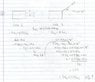# Kinetic Energy and Work energy theorem

• jasonbans

## Homework Statement

A toboggan is initially moving at a constant velocity along a snowy horizontal
surface where friction is negligible. When a pulling force is applied parallel to
the ground over a certain distance, the kinetic energy increases by 47%. By what
percentage would the kinetic energy have changed if the pulling force had been
at an angle of 38° above the horizontal?

w= Fd cos theta
w= 1/2mv^2

## The Attempt at a Solution

so Ek = 1.47 Ek intial Ek = kinetic energy

37%?

yeah can you explain what you did?

First find an expression for the change in KE when the force is applied along the horizontal.

you mean a expression for both cases?
we know that in the first case the force and displacement act in the same direction meaning no work is done.
and the second case i don't get, how can you get W = (Fcos38)d where F and d is not even given

we know that in the first case the force and displacement act in the same direction meaning no work is done.
and the second case i don't get, how can you get W = (Fcos38)d where F and d is not even given

Work is done in BOTH cases. This work results in an increase in KE.
No more numerical data is necessary. Just assume initial velocity is u in both cases and final is V for the first case and v for the second (symbols chosen so that big V is for the greater value and small v for the smaller value - I hate to call them v1 and v2!) since the final will be different for the two cases.

how am i suppose to find the velocity if they give so little information?

Assume that applied force is F in first case and Fcos38 in the second case.
Initial velocity is u in both.
Distance traveled is assumed to be constant e.g. x in both.

What is V in terms of u, x and acceleration?

What is V in terms of u, x and acceleration?

i did this instead , this should make sense right? cause the change in W = the final kinetic energy - the intial kinetic energy, and W = Fd in first case and second is W=fdcos38

#### Attachments

•img103.jpg
41.3 KB · Views: 373
I think it is OK.